Technical Article

# Using the Noise Figure Metric to Analyze Noise in RF Circuits

December 16, 2022 by Dr. Steve Arar

## Concerning noise analysis in RF analog design, learn about the noise figure metric, including key aspects of this specification through examples.

Aside from some specific applications, for example, when a dithering effect is needed, noise is generally an unwanted phenomenon. Scientists and engineers have characterized the noise produced by different circuit components and developed methods that can be used to analyze the noise performance of a circuit. In analog circuit design, we normally model the noise effect as input-referred noise voltage and current sources. However, in radio frequency (RF) design, the noise figure metric can be a more useful way of characterizing the noise performance of a circuit.

In this article, we’ll introduce the noise figure metric, highlight some subtleties of this specification, and finally take a look at an example to clarify the discussed concepts.

### Noise Analysis in RF Analog Design

We normally model the effect of all noise sources in the circuit by a series voltage source ($$\overline{v^{2}_{n,input}}$$) and a parallel current source ($$\overline{i^{2}_{n,input}}$$), as illustrated in Figure 1.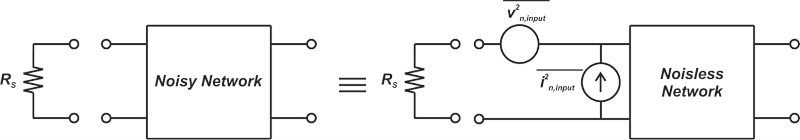##### Figure 1. Effect of noise sources in a circuit with a series voltage source and parallel current source.

In Figure 1, the “noiseless network” is the same as the “noisy network” except that its components (resistors, transistors, etc.) are assumed to be noise-free. By equating the output noise of the two circuits, we can find the value of the input-referred noise voltage and current sources. The two input generators are sufficient and necessary to correctly model the noise of a linear two-port network for any source impedance (RS).

### Understanding Source Impedance Using OP27 Op-amp Example

In RF design, especially discrete RF design, we normally prefer to use the noise figure concept rather than the noise voltage and current sources model. However, the above model can help us better understand noise behavior in electronic circuits and the subtleties of the noise figure metric.

One key observation is that the output noise of the circuit depends on the output impedance of the preceding stage (or the source impedance, RS). This can be understood by considering the extreme cases of R= 0 and RS tending to infinity (R→ ∞). For R= 0, $$\overline{i^{2}_{n,input}}$$ is shorted out and has no effect on the output noise.

On the other hand, if RS tends to infinity, $$v^{2}_{n,input}$$ cannot produce output noise. Figure 2 shows the effect of source resistance (R) on a real-world op-amp (OP27) configuration.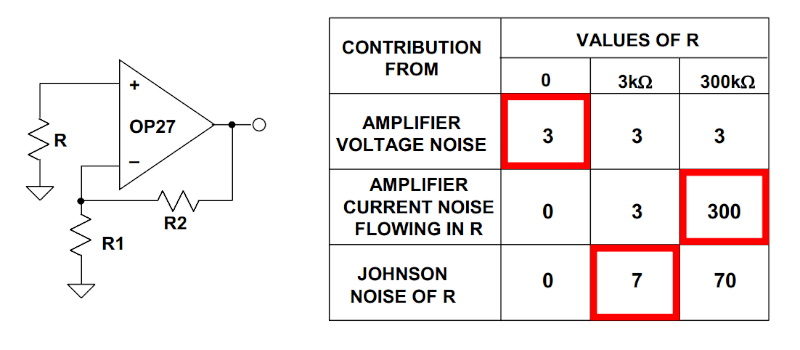##### Figure 2. Effect of source resistance on an OP27 op-amp configuration. Image used courtesy of Analog Devices

The OP27 has a voltage noise of $$3\frac{nV}{\sqrt{Hz}}$$ and current noise of $$1\frac{pA}{\sqrt{Hz}}$$.

In the above example, only the noise from the source impedance (R) and the op-amp are considered. For R = 0, the op-amp noise current source is shorted out, and the overall input referred-noise is determined by the voltage noise of the op-amp $$3\frac{nV}{\sqrt{Hz}}$$.

When we increase the source resistance to R = 3 kΩ and 300 kΩ, the op-amp noise current source is expected to flow through both the source impedance and the input impedance of the op-amp. However, since the op-amp input impedance is in the range of several MΩ, the noise current still flows mainly through the source impedance. As a result, for R = 3 kΩ and 300 kΩ, the noise current produces an equivalent noise voltage of $$3\frac{nV}{\sqrt{Hz}}$$ and 300 $$\frac{nV}{\sqrt{Hz}}$$, respectively.

For R = 3 kΩ and 300 kΩ, the noise voltage of the op-amp still produces $$3\frac{nV}{\sqrt{Hz}}$$ at the op-amp input because these resistor values are relatively small compared with the op-amp input impedance.

The table in Figure 2 also gives the thermal noise of R for the three different values considered. As can be seen, the dominant noise source (highlighted by red rectangles in Figure 2's table) and, consequently, the overall noise performance of the circuit change with the source impedance. This is an important concept to remember when using the noise figure metric (as will be discussed shortly).

### The Need for an Alternative Noise Metric

The input-referred noise generators model doesn’t lend itself to RF design. For example, when calculating the input-referred noise current of an RF gain stage, we need to measure the trans-impedance of the stage at the frequency of interest. Such measurements are quite challenging at high frequencies. Therefore, it is desirable to find another noise performance metric that can be more easily measured at high frequencies.

Besides, in many RF systems, we are interested in the ratio of signal power to noise power (SNR or signal-to-noise ratio). The SNR specifies the quality of the signal and ultimately determines how many of the digital bits our communication system receives will be in error (a bit error rate of the system).

The required SNR depends on various factors, such as:

• Modulation scheme
• Bit rate
• Energy per bit
• Filter bandwidth

Most digital communication systems need at least 10 dB of SNR. The SNR is measured at the output of the receiver system, where the demodulation is performed. As shown in Figure 3, a typical receiver signal chain consists of several different blocks, such as the low-noise amplifier (LNA), mixer, filter, and analog-to-digital converter (ADC).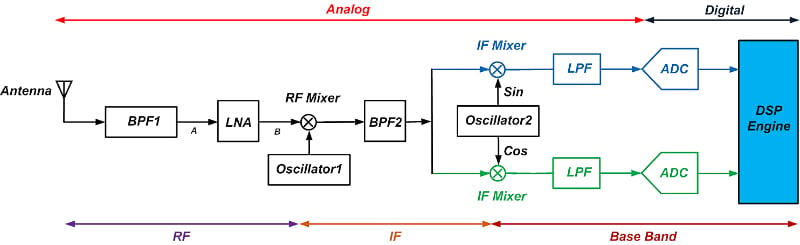##### Figure 3. An example receiver signal chain block diagram.

RF engineers need to know how each of these circuit components contributes to the noise performance and how the SNR is degraded as the signal travels through the signal chain. Therefore, a noise performance metric directly related to SNR can be more helpful in an RF design. This is where the noise figure specification stands out since the noise figure can be more easily measured at high frequencies and is defined directly based on the SNR specification.

### Noise Factor and Noise Figure Measurement

The noise factor (F) of a circuit is defined as the ratio of the SNR at the input to the SNR at the output:

$F=\frac{SNR_{in}}{SNR_{out}}$

##### Equation 1.

In the above equation, the noise and signal power quantities are expressed in linear terms rather than in decibels. The noise factor expressed in decibels is called noise figure (NF):

$NF=10log_{10} \big( \frac{SNR_{in}}{SNR_{out}} \big)$

##### Equation 2.

It should be noted that some references don’t make this distinction and use the term noise figure to refer to both Equations 1 and 2. In this case, we should determine from the context whether the noise figure is expressed in decibels or linear terms.

For a noiseless circuit, the input and output SNR is the same, leading to F = 1 and NF = 0 dB. The noise figure is a direct measure of the SNR degradation caused by the circuit. For example, if the SNR at the input of a circuit is 60 dB and the circuit noise figure is 7 dB, then the SNR at the output of the circuit is 53 dB. To be more precise, this statement is valid, assuming that the input noise power of the circuit is equal to the reference noise power at which the circuit's NF is calculated (we'll discuss this in great detail in an upcoming article).

### Other Noise Figure Definitions

Using a little algebra, we can derive useful alternative expressions from Equation 1. If we denote the signal power at the input and output of the circuit by Si and So, and the noise power at the input and output by Ni and No, we obtain the following equation:

$F=\frac{\frac{S_i}{N_i}}{\frac{S_o}{N_o}}=\frac{S_i}{N_i} \times \frac{N_o}{S_o}$

With that in mind, you might wonder, how is the output signal related to the input signal? Since these are power quantities, we need to consider the power gain of the circuit. With a power gain of G, we have S= GSi, which leads to Equation 3:

$F= \frac{N_o}{GN_i}$

##### Equation 3.

In Equation 3, No is the total noise at the output. It includes the effect of the internal noise sources within the circuit and the noise from the source impedance (or the output impedance of the preceding stage). Ni is the noise the source impedance (RS) produces at the circuit's input. From Equation 3, the noise factor is the total output noise divided by that part of the output noise that comes from the source resistance.

To derive another expression, let’s focus on the part of the output noise that stems from the source impedance, No(source), and the part that is produced by the internal noise sources within the circuit No(added). Therefore, we get:

$N_{o(source)}=GN_{i}$

$N_{o}=N_{o(source)}+N_{o(added)}$

Substituting these equations into Equation 3 produces Equation 4:

$F=1+\frac{N_{o(added)}}{N_{o(source)}}$

##### Equation 4.

Equation 4 shows that the value we obtain for the noise figure depends on the source impedance (RS) connected to the circuit. In Equation 4, No(source) is obviously a function of RS. However, based on our discussion in the previous section, we know that the noise of the circuit itself is also a function of RS. Therefore, the noise figure must be specified concerning a known source impedance—typically 50 Ω.

### Calculating the Noise Figure in an Example Circuit

In this example, we’ll use Equations 1 and 4 to calculate the noise figure of the following circuit (Figure 4).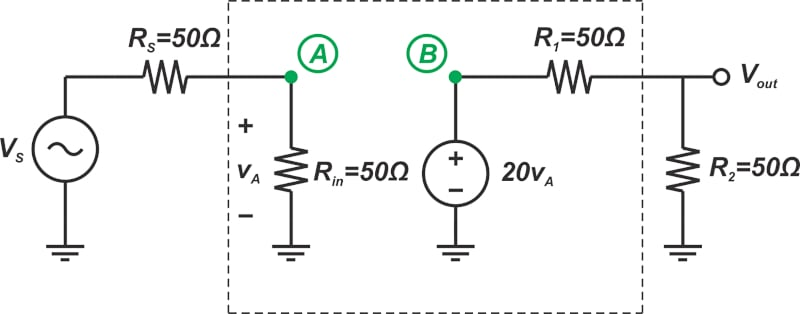##### Figure 4. Example circuit.

We assume that the RMS value of the input voltage is VS, all resistor values are 50 Ω, and the noise bandwidth of the system is B= 1 MHz.

To use Equation 1, we need to calculate the SNR at the circuit input and output. Since R= Rin, the input signal is halved at the circuit's input (node A). Therefore, the RMS value of the signal at node A is:

$V_A=\frac{V_s}{2}$

The input signal power is found as:

$S_i=\frac{V_A^2}{R_{in}}=\frac{V_s^2}{4R_{in}}$

##### Equation 5.

With that done, we might ask how we can calculate the input noise. We know that the RMS noise voltage of a resistor R in $$V/\sqrt{Hz}$$ is given by:

$V_{n, rms}=\sqrt{4kTR}$

Figure 5 shows the circuit diagram for calculating the noise from RS.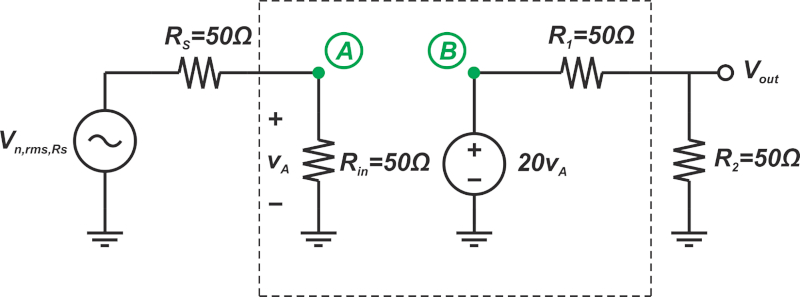##### Figure 5. Example circuit diagram for calculating noise from RS.

In this diagram, the input voltage source is shorted out, and the noise voltage of RS is added in series with this resistor. Since R= Rin, the noise voltage of RS is halved at the input, producing an input noise power of:

$N_i=\frac{(Noise \text{ } Voltage \text{ } @ \text{ } Input \text{ } Due \text{ }to \text{ } R_S)^2}{R_{in}^2}=\frac{V_{n,rms}^2}{4R_{in}}=kT$

##### Equation 6.

Ni is the noise power at the circuit input. It should be noted that Ni is calculated assuming that the input impedance of the circuit (Rin) is noiseless. Since Vn,rms is in $$V/\sqrt{Hz}$$, the above equation gives the noise power per unit bandwidth $$(V^{2}/Hz)$$.

Therefore, the result of Equation 6 should be multiplied by the noise bandwidth Bn to find the total noise power over the bandwidth of interest. Taking the bandwidth into account, Equations 5 and 6 give us the input SNR:

$SNR_{in}=\frac{V_S^2}{4kTB_nR_{in}}$

##### Equation 7.

To calculate the output signal and noise power, we need to find the power gain of the circuit (the ratio of output power to input power):

$G=\frac{P_{out}}{P_{in}}=\frac{\frac{V_{out}^2}{R_2}}{\frac{V_A^2}{R_{in}}}=\frac{V_{out}^2}{V_A^2}$

##### Equation 8.

In the above equation, Vout denotes the RMS of the output voltage. From the circuit diagram, we note that:

$V_{out}=V_A \times 20 \times \frac{R_2}{R_2+R_1}=10V_A$

Equation 8 simplifies to G=100. The output signal power is calculated as:

$S_o=GS_i=100\times \frac{V_s^2}{4R_{in}}$

##### Equation 9.

From Equation 6, the output noise due to the source resistance is calculated as:

$N_{o(source)}=GN_i=100kT$

##### Equation 10.

Figure 6 shows the circuit diagram for calculating the noise contribution of Rin.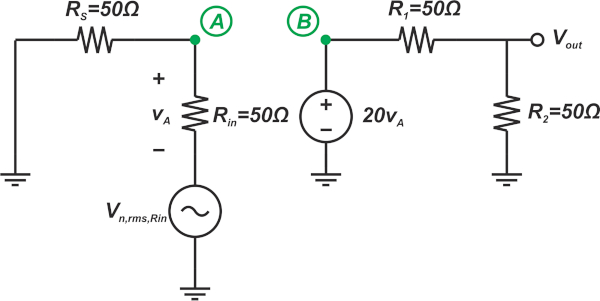##### Figure 6. Example circuit diagram for calculating the noise contribution of Rin.

Comparing the above diagram with Figure 5, we can conclude that the output noise from Rin is the same as that from RS (100 kT). The output noise power from each R1 and R2 can be easily calculated as kT. Therefore, the total output noise is:

$N_{o}= N_{o, RS}+N_{o, Rin}+N_{o, R1}+N_{o,R2}=100kT+100kT+kT+kT=202kT$

##### Equation 11.

Again note that Equations 10 and 11 give the noise power per unit bandwidth (V2/Hz), and the result should be multiplied by the noise bandwidth Bn to find the total noise power over the bandwidth of interest. Equations 9 and 11 give us the output SNR:

$SNR_o=\frac{S_o}{N_o}=\frac{100V_s^2}{808kTB_nR_{in}}$

##### Equation 12.

Finally, from Equations 7 and 12, the noise factor is calculated:

$F=\frac{SNR_{in}}{SNR_{out}}=\frac{V_S^2}{4kTB_nR_{in}}\times \frac{808kTB_nR_{in}}{100V_s^2}=2.02$

##### Equation 13.

Expressed in decibels, we obtain NF = 3.05 dB. Now, let’s use Equation 4 to analyze the circuit noise figure. In this case, we only need to calculate the part of the output noise that stems from the source impedance No(source) and the part that is produced by the circuit No(added). No(added) can be calculated from Equations 10 and 11:

$N_{o(added)}= N_{o}-N_{o(source)}=202kT-100kT=102kT$

##### Equation 14.

Substituting Equations 10 and 14 into Equation 4 yields:

$F=1+\frac{N_{o(added)}}{N_{o(source)}}=1+\frac{102kT}{100kT}=2.02$

that is consistent with the previous calculations. With Equation 4, we don’t need to calculate the input and output signal power as well as the input and output SNR. As a result, Equation 4 can be more easily calculated.

Featured image used courtesy of Adobe Stock